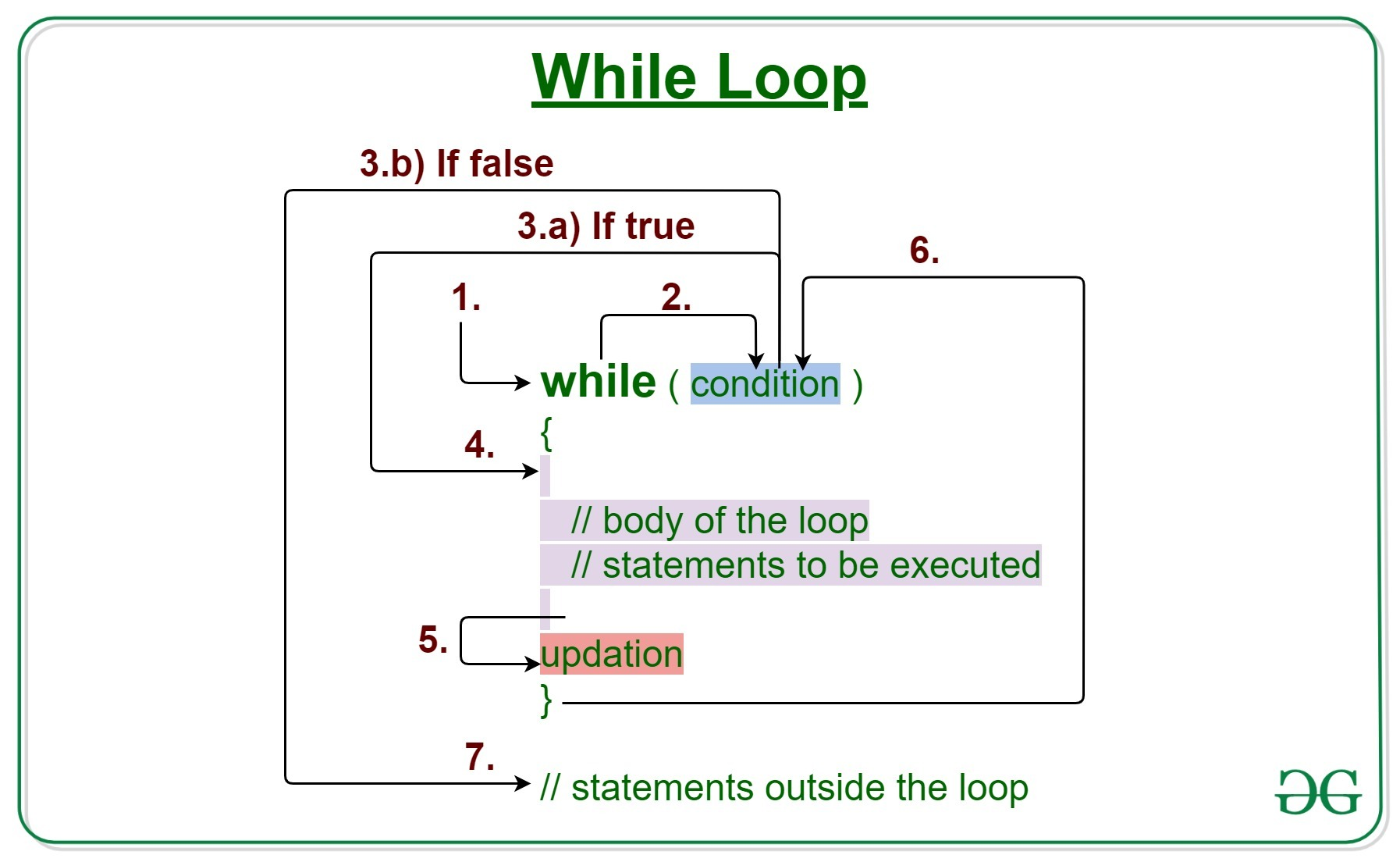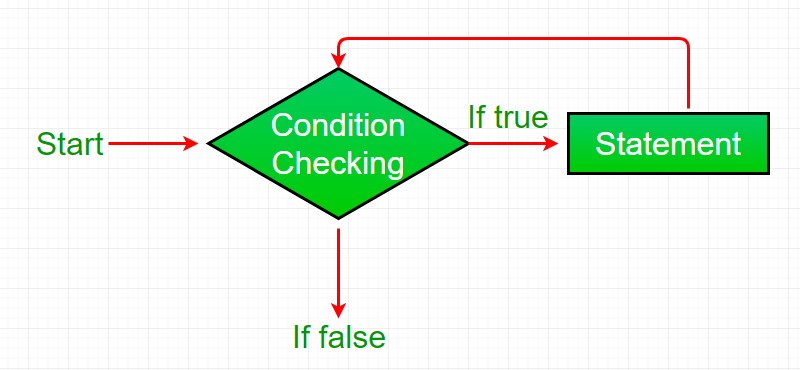Related Articles

# Java while loop with Examples

• Difficulty Level : Easy
• Last Updated : 17 Feb, 2021

Loops in Java come into use when we need to repeatedly execute a block of statements.

Java while loop is a control flow statement that allows code to be executed repeatedly based on a given Boolean condition. The while loop can be thought of as a repeating if statement.Syntax:

```while (test_expression)
{
// statements

update_expression;
}```

The various parts of the While loop are:

1. Test Expression: In this expression we have to test the condition. If the condition evaluates to true then we will execute the body of the loop and go to update expression. Otherwise, we will exit from the while loop.
Example:
`i <= 10`
2. Update Expression: After executing the loop body, this expression increments/decrements the loop variable by some value.
Example:
`i++;`

How does a While loop executes?

1. Control falls into the while loop.
2. The flow jumps to Condition
3. Condition is tested.
1. If Condition yields true, the flow goes into the Body.
2. If Condition yields false, the flow goes outside the loop
4. The statements inside the body of the loop get executed.
5. Updation takes place.
6. Control flows back to Step 2.
7. The do-while loop has ended and the flow has gone outside.

Flow chart while loop (for Control Flow):Example 1: This program will try to print “Hello World” 5 times.

 `// Java program to illustrate while loop.`` ` `class` `whileLoopDemo {``    ``public` `static` `void` `main(String args[])``    ``{``        ``// initialization expression``        ``int` `i = ``1``;`` ` `        ``// test expression``        ``while` `(i < ``6``) {``            ``System.out.println(``"Hello World"``);`` ` `            ``// update expression``            ``i++;``        ``}``    ``}``}`
Output:
```Hello World
Hello World
Hello World
Hello World
Hello World
```

Dry-Running Example 1: The program will execute in the following manner.

```1. Program starts.
2. i is initialized with value 1.
3. Condition is checked. 1 < 6 yields true.
3.a) "Hello World" gets printed 1st time.
3.b) Updation is done. Now i = 2.
4. Condition is checked. 2 < 6 yields true.
4.a) "Hello World" gets printed 2nd time.
4.b) Updation is done. Now i = 3.
5. Condition is checked. 3 < 6 yields true.
5.a) "Hello World" gets printed 3rd time
5.b) Updation is done. Now i = 4.
6. Condition is checked. 4 < 6 yields true.
6.a) "Hello World" gets printed 4th time
6.b) Updation is done. Now i = 5.
7. Condition is checked. 5 < 6 yields true.
7.a) "Hello World" gets printed 5th time
7.b) Updation is done. Now i = 6.
8. Condition is checked. 6 < 6 yields false.
9. Flow goes outside the loop. Program terminates.
```

Example 2: This program will find the summation of numbers from 1 to 10.

 `// Java program to illustrate while loop`` ` `class` `whileLoopDemo {``    ``public` `static` `void` `main(String args[])``    ``{``        ``int` `x = ``1``, sum = ``0``;`` ` `        ``// Exit when x becomes greater than 4``        ``while` `(x <= ``10``) {``            ``// summing up x``            ``sum = sum + x;`` ` `            ``// Increment the value of x for``            ``// next iteration``            ``x++;``        ``}``        ``System.out.println(``"Summation: "` `+ sum);``    ``}``}`
Output:
```Summation: 55
```

Related Articles:

Attention reader! Don’t stop learning now. Get hold of all the important Java Foundation and Collections concepts with the Fundamentals of Java and Java Collections Course at a student-friendly price and become industry ready. To complete your preparation from learning a language to DS Algo and many more,  please refer Complete Interview Preparation Course.

My Personal Notes arrow_drop_up